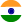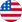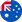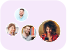24*7 support on WhatsAppChat NowProduct Edition:1st Edition
Author: A. K. Nandakumaran
Book Name: Ordinary Differential Equations: Principles and Applications
Subject Name: Maths

# Ordinary Differential Equations: Principles and Applications 1st Edition Solutions

0 out of 5.0
35 reviews23 Students
have requested for homework help from this book

Written in a clear, logical and concise manner, this comprehensive resource allows students to quickly understand the key principles, techniques and applications of ordinary differential equations. Important topics including first and second order linear equations, initial value problems and qualitative theory are presented in separate chapters. The concepts of two point boundary value problems, physical models and first order partial differential equations are discussed in detail. The text uses tools of calculus and real analysis to get solutions in explicit form. While discussing first order linear systems, linear algebra techniques are used. The real-life applications are interspersed throughout the book to invoke reader's interest. The methods and tricks to solve numerous mathematical problems with sufficient derivations and explanation are provided. The proofs of theorems are explained for the benefit of the readers.

5
18
4
7
3
2
2
7
1
1

0

## Students who viewed this book also checked out